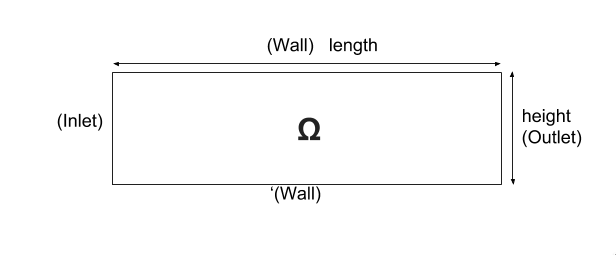# Mass transport in a Stokes flow in a pipe

## 1. Running the model

The command line to run this pipestokes case

``mpirun -np 4 feelpp_toolbox_fluid --case "github:{repo:toolbox,path:examples/modules/heatfluid/examples/pipestockes_mass}"``

## 3. Geometry

### 3.1. Model & Toolbox

We consider a 2D model representative of a laminar incompressible flow around an obstacle. The flow domain, named $\Omega_f$, is contained into the rectangle $\lbrack 0,Long \rbrack \times \lbrack 0,Haut \rbrack$. It is characterised, in particular, by its dynamic viscosity $\mu_f$ and by its density $\rho_f$.The goal of this benchmark is to couple the Stockes equations and the Concentration equations.
we remind that the Stokes equation are

\left\{ \begin{aligned} \boldsymbol{\mu}\boldsymbol{\Delta u}+\boldsymbol{\nabla p}=f\\ \boldsymbol{\nabla}\cdot\boldsymbol{u}=0 \end{aligned} \right.

with $\boldsymbol{\mu}$ is the dynamic viscosity, $\boldsymbol{p}$ is the pressure ,$f$ the source and u the velocity.

And the Concentration equations is

$\begin{array}[c]{rl} \frac{\partial C}{\partial t} + \boldsymbol{u} \cdot \nabla C - \nabla \cdot \left( D_{p} \nabla C \right) = 0, \quad \text{ in } \Omega \end{array}$

With $D_{p}$ the diffusion coefficient on the plasma.

We used the heat fluid toolbox, we replaced the temperature by the Concentration, k by $D_{p}$, and we posed $\rho C_{p}=1$ to have the same kind of equations.

## 4. Input parameters

The following table displays the various fixed and variables parameters of this test-case.

 Name Description Units $u$ fluid velocity $m/s$ $\rho$ density of the fluid $kg/m^3$ $\nu$ dynamic viscosity $kg/(m×s)$ $p$ pression $Pa$ $f$ source term $kg/(m^3×s)$ $C_p$ thermal capacity $J/(kg∗K)$ $T$ Temperature $K$ $Q$ heat source $W.m^{-3}$ $D_{p}$ the diffusion coefficient on the plasma $\mu m²/s$

### 4.1. initial condition

• For the fluid:

We use a parabolic velocity profile, in order to describe the flow inlet by $\Gamma_{in}$, which can be express by

$v_{inlet}=D y(height-y)$

To determine $D$, we know that for $y=\frac{height}{2}$ we have the maximal velocity, so

$D=\frac{v_{max}}{\frac{height}{2}\left(height-\frac{height}{2}\right)}$
• For the Concentration:

We give as source this Concentration

$C_{in}=300*(y>0.15)*(y<0.5)+(293.15*(y<(0.15-1e-9)))+(293.15*(y>(0.5-1e-9)))$

### 4.2. Materials

```   "Materials":
{
"Fluid":{
"rho":"1.0",
"mu":"1.0",
"k":"2400.e-6",
"Cp":"1.0"

}
}```

### 4.3. Boundary conditions

For the fluid:

We set

• On $\Gamma_{in}$, an inflow Dirichlet condition : $\boldsymbol{u}_f=(v_{in},0)$

• On $\Gamma_{wall}$ and $\Gamma_{obst}$, a homogeneous Dirichlet condition : $\boldsymbol{u}_f=\boldsymbol{0}$

• On $\Gamma_{out}$, a Neumann condition : $\boldsymbol{\sigma}_f\boldsymbol{ n }_f=\boldsymbol{0}$

For the Concentration:

• On $\Gamma_{in}$, an inflow Dirichlet condition : $\boldsymbol{C}_f=C_{in}$

```     "BoundaryConditions":
{
"velocity":
{
"Dirichlet":
{
"inlet":
{
"expr":"{D*y*(height-y),0}:y:height:D"
},
"wall1":
{
"expr":"{0,0}"
},
"wall2":
{
"expr":"{0,0}"
}
}
},
"fluid":
{
"outlet":
{
"outlet":
{
"expr":"0"
}
}
},
"temperature":
{
"Dirichlet":
{
"inlet":
{
"expr":"300*(y>0.15)*(y<0.5)+(293.15*(y<(0.15-1e-9)))+(293.15*(y>(0.5-1e-9))):y"
}
}
}
}```

### 4.4. Fields

We are intersting in the visualisation of the three fields : the velocity, the pressure and the temperature of the fluid

```    "Exports":
{
"fields":["fluid.velocity","fluid.pressure","heat.temperature","fluid.pid"]
}```

### 4.5. Measures

the pressure is measured on two points to see the behavior of the pressure as a function of time

```          "Measures":
{
"Forces":"wall2",
"Points":
{
"pointA":
{
"coord":"{0.6,0.2,0}",
"fields":"pressure"
},
"pointB":
{
"coord":"{0.15,0.2,0}",
"fields":"pressure"
}
}
}```

## 5. Numerical Experiments

We run this model, using the command labeled at the top, we have the following results.

For The temperature: